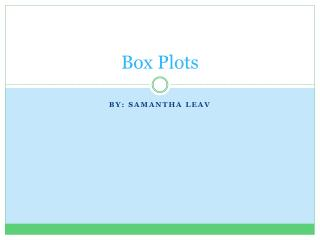# Box Plots - PowerPoint PPT PresentationDownload PresentationBox Plots

Box Plots
Download Presentation## Box Plots

- - - - - - - - - - - - - - - - - - - - - - - - - - - E N D - - - - - - - - - - - - - - - - - - - - - - - - - - -
##### Presentation Transcript

1. Box Plots By: Samantha Leav

2. Common Core Standards S-ID1. Represent data with plots on the real number line (dot plots, histograms, and box plots)

3. What is a box plot? • A box plot (also known as a box-and-whisker diagram) is a convenient way to graphically depict groups of numerical data with their five-number summaries: • Minimum • Lower Quartile • Median • Upper Quartile • Maximum

4. Five-Number Summaries Minimum (min) – Smallest value of the data set Median (med) – The middle value of the data set. Maximum (max) – Largest value of the data set Quartiles – There are three points that divide a data set into 4 equal parts. Each point is a quartile. Each quartile represents 25% of the data. Lower Quartile (Q1 – 25%) – This is the point that is between the minimum and median. (In other words the median from the minimum to the median) Upper Quartile (Q3 – 75%) – This is the point that is between that median and maximum. (In other words the median from the median to the maximum) Where is Q2 and Q4?

5. Example of Box Plot

6. Steps to finding five-number summary • Put your data points into order from least to greatest. • The minimum value is the smallest value. • The maximum value is the largest value. • Cross values out from the smallest values and greatest values until you get one or two values left in the middle. • If you have one value left this is your median. • If you get two values, add them and divide by two and this will be your median. • To find the quartiles we find the median of the first half of data points and then do the same to the second half of the data points. (When finding these do not include the median value) • We do the same thing we did with the whole data sets median if we have one or two values left

7. Let’s try it together. Even Amount of Numbers Odd Amount of Numbers • 2, 6, 7, 10, 14, 15 • Min? • Max? • Median? • Lower quartile? • Upper quartile? • 1, 4, 9, 12, 16, 23, 24 • Min? • Max? • Median? • Lower quartile? • Upper quartile?

8. How to create a box plot • Now, we have all of the necessary information to create a box plot. • Draw a scale (number line) above which the plot will be drawn • Include numbers and units • Can be vertical or horizontal • Draw rectangular box with ends at quartiles • Height not important • Draw line through box at median • Draw two “whiskers” from corresponding ends of box to extreme values • Label with 5 numbers

9. Let’s draw a box plot for our two sets of data. Recall what a box plot looks like

10. Try this on your own. Find the five-number summary and create a box plot. 7, 9, 1, 3, 7, 5, 2, 4, 6, 10, 1

11. Some fun with the calculators. We will be using our calculators and CBR’s to collect data. After we’ve obtained the data we will find the five-number summary and then create a box plot.